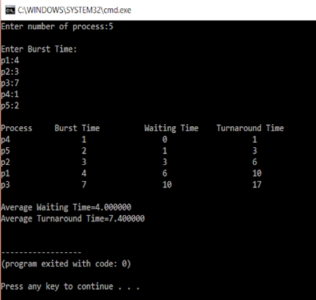# Shortest Job First Scheduling in C Programming

Published on Aug 30,2019 12.6K ViewsShortest job first(SJF) is a scheduling algorithm, that is used to schedule processes in an operating system. It is a very important topic in Scheduling when compared to round-robin and FCFS Scheduling. In this article, we will discuss the Shortest Job First Scheduling in the following order:

There are two types of SJF

• Pre-emptive SJF

• Non-Preemptive SJF

These algorithms schedule processes in the order in which the shortest job is done first. It has a minimum average waiting time.

There are 3 factors to consider while solving SJF, they are

1. BURST Time
2. Average waiting time
3. Average turnaround time

Non-Preemptive Shortest Job First

Here is an example

 Processes Id Burst Time Waiting Time Turn Around Time 4 3 0 3 1 6 3 9 3 7 9 16 2 8 16 25

Average waiting time = 7

Average turnaround time = 13

T.A.T= waiting time + burst time

Code for Shortest Job First Scheduling

```#include<stdio.h>
int main()
{
int bt,p,wt,tat,i,j,n,total=0,pos,temp;
float avg_wt,avg_tat;
printf("Enter number of process:");
scanf("%d",&n);

printf("nEnter Burst Time:n");
for(i=0;i<n;i++)
{
printf("p%d:",i+1);
scanf("%d",&bt[i]);
p[i]=i+1;
}

//sorting of burst times
for(i=0;i<n;i++)
{
pos=i;
for(j=i+1;j<n;j++)
{
if(bt[j]<bt[pos])
pos=j;
}

temp=bt[i];
bt[i]=bt[pos];
bt[pos]=temp;

temp=p[i];
p[i]=p[pos];
p[pos]=temp;
}

wt=0;

for(i=1;i<n;i++)
{
wt[i]=0;
for(j=0;j<i;j++)
wt[i]+=bt[j];

total+=wt[i];
}

avg_wt=(float)total/n;
total=0;

printf("nProcesst    Burst Time    tWaiting TimetTurnaround Time");
for(i=0;i<n;i++)
{
tat[i]=bt[i]+wt[i];
total+=tat[i];
printf("np%dtt  %dtt    %dttt%d",p[i],bt[i],wt[i],tat[i]);
}

avg_tat=(float)total/n;
printf("nnAverage Waiting Time=%f",avg_wt);
printf("nAverage Turnaround Time=%fn",avg_tat);
}```

Output:In the above program, we calculate the average waiting and average turn around times of the jobs. We first ask the user to enter the number of processes and store it in n. We then accept the burst times from the user. It is stored in the bt array.

After this, the burst times are sorted in the next section so the shortest one can be executed first. Here selection sort is used to sort the array of burst time bt.

Waiting time of the first element is zero, the remaining waiting time is calculated by using two for loop that runs from 1 to in that controls the outer loop and the inner loop is controlled by another for loop that runs from j=0 to j<i.  Inside the loop, the waiting time is calculated by adding the burst time to the waiting time.

```for(i=1;i<n;i++)
{
wt[i]=0;
for(j=0;j<i;j++)
wt[i]+=bt[j];
total+=wt[i];
}```

Total is the addition of all the waiting time together. The average waiting time is calculated:

avg_wt=(float)total/n;

and it is printed.

Next, the turnaround time is calculated by adding the burst time and the waiting time

```for(i=0;i<n;i++)
{
tat[i]=bt[i]+wt[i];
total+=tat[i];
printf("np%dtt  %dtt    %dttt%d",p[i],bt[i],wt[i],tat[i]);
}```

Again, here the for loop is used. And the total variable here holds the total turnaround time. After this the average turnaround time is calculated. This is how Non-Preemptive scheduling takes place

Code for Pre-emptive SJF Scheduling

```#include <stdio.h>

int main()
{
int arrival_time, burst_time, temp;
int i, smallest, count = 0, time, limit;
double wait_time = 0, turnaround_time = 0, end;
float average_waiting_time, average_turnaround_time;
printf("nEnter the Total Number of Processes:t");
scanf("%d", &limit);
printf("nEnter Details of %d Processesn", limit);
for(i = 0; i < limit; i++)
{
printf("nEnter Arrival Time:t");
scanf("%d", &arrival_time[i]);
printf("Enter Burst Time:t");
scanf("%d", &burst_time[i]);
temp[i] = burst_time[i];
}
burst_time = 9999;
for(time = 0; count != limit; time++)
{
smallest = 9;
for(i = 0; i < limit; i++)
{
if(arrival_time[i] <= time && burst_time[i] < burst_time[smallest] && burst_time[i] > 0)
{
smallest = i;
}
}
burst_time[smallest]--;
if(burst_time[smallest] == 0)
{
count++;
end = time + 1;
wait_time = wait_time + end - arrival_time[smallest] - temp[smallest];
turnaround_time = turnaround_time + end - arrival_time[smallest];
}
}
average_waiting_time = wait_time / limit;
average_turnaround_time = turnaround_time / limit;
printf("nnAverage Waiting Time:t%lfn", average_waiting_time);
printf("Average Turnaround Time:t%lfn", average_turnaround_time);
return 0;
}```

Output:The only difference in preemptive and non-preemptive is that when two burst times are same the algorithm evaluates them on first come first serve basis. Hence there is an arrival time variable.

With this, we come to an end of this Shortest Job Scheduling in C article. I hope you got an idea of how this scheduling works.

Now that you have understood the basics of SJF Scheduling in C, check out the training provided by Edureka on many technologies like Java, Spring and  many more, a trusted online learning company with a network of more than 250,000 satisfied learners spread across the globe

Got a question for us? Mention it in the comments section of this “Shortest Job First Scheduling in C Programming” blog and we will get back to you as soon as possible.